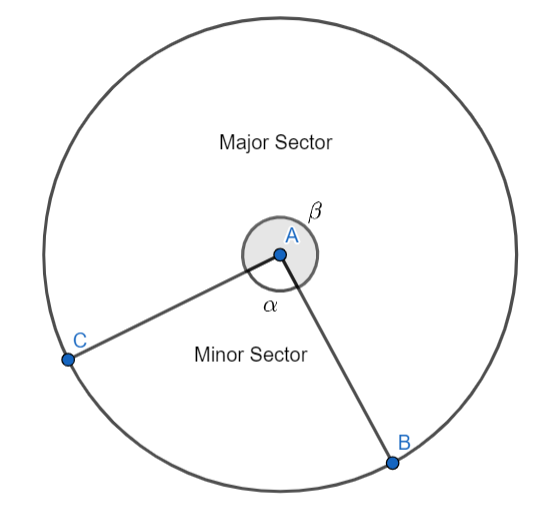Courses
Courses for Kids
Free study material
Free LIVE classes
MoreLIVE
Join Vedantu’s FREE Mastercalss

# Formula to find the area of the quadrant of a circle is:A.$\pi {{r}^{2}}$ B.$\dfrac{\pi {{r}^{2}}}{2}$ C.$\dfrac{\pi {{r}^{2}}}{4}$ D.$\dfrac{\pi {{r}^{2}}}{8}$Verified
362.1k+ views
Hint: The given problem is related to the area of a circle. The area of a sector of a circle, having radius $r$, which subtends an angle $\theta$ at the centre is given as $\left( \dfrac{\theta }{2\pi } \right)\times \pi {{r}^{2}}$ . The angle subtended by the quadrant of a circle at the centre is $\dfrac{\pi }{2}$ .

Before we proceed with the solution, we must understand the sector of a circle. The sector of a circle is defined as the region enclosed between two radii and the arc of the circle. If the angle between the radii is $\theta$ , then $\theta$ is called the central angle. The region corresponding to the smaller central angle is called the minor sector and the region corresponding to the larger central angle is called the major sector. If $\theta =\dfrac{\pi }{2}$ , then the sector is called a quadrant.Consider the figure. In the figure $\alpha <\beta$ . So, the sector corresponding to angle $\alpha$ is the minor sector and the sector corresponding to angle $\beta$ is the major sector.
Now, the area of a sector of a circle, having radius $r$ , which subtends an angle $\theta$ at the centre is given as $\left( \dfrac{\theta }{2\pi } \right)\times \pi {{r}^{2}}$ . We know the angle subtended by the quadrant of a circle at the centre is $\dfrac{\pi }{2}$ . So, the area of the quadrant will be equal to $\dfrac{\dfrac{\pi }{2}}{2\pi }\times \pi {{r}^{2}}=\dfrac{\pi }{4\pi }\pi {{r}^{2}}=\dfrac{\pi {{r}^{2}}}{4}$ .
Hence, the area of the quadrant of a circle having radius $r$ is equal to $\dfrac{\pi {{r}^{2}}}{4}$ .